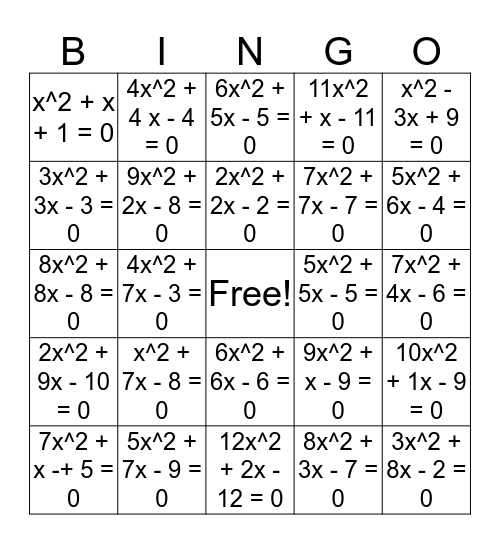# QuadraticsThis bingo card has a free space and 24 words: x^2 + 7x - 8 = 0, 5x^2 + 7x - 9 = 0, 2x^2 + 2x - 2 = 0, 7x^2 + x -+ 5 = 0, x^2 - 3x + 9 = 0, x^2 + x + 1 = 0, 3x^2 + 3x - 3 = 0, 4x^2 + 4 x - 4 = 0, 5x^2 + 5x - 5 = 0, 6x^2 + 6x - 6 = 0, 7x^2 + 7x - 7 = 0, 8x^2 + 8x - 8 = 0, 9x^2 + x - 9 = 0, 10x^2 + 1x - 9 = 0, 9x^2 + 2x - 8 = 0, 8x^2 + 3x - 7 = 0, 7x^2 + 4x - 6 = 0, 6x^2 + 5x - 5 = 0, 5x^2 + 6x - 4 = 0, 4x^2 + 7x - 3 = 0, 3x^2 + 8x - 2 = 0, 2x^2 + 9x - 10 = 0, 11x^2 + x - 11 = 0 and 12x^2 + 2x - 12 = 0.

## Play Online

Share this URL with your players:For more control of your online game, create a clone of this card first.

## Probabilities

With players vying for a you'll have to call about __ items before someone wins. There's a __% chance that a lucky player would win after calling __ items.

Tip: If you want your game to last longer (on average), add more unique words/images to it.